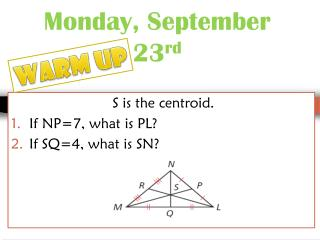Download PresentationMonday, September 23 rd

# Monday, September 23 rd - PowerPoint PPT Presentation

Monday, September 23 rd. Warm Up. S is the centroid. If NP=7, what is PL? If SQ=4, what is SN ?. Week at a Glance. Monday: Review-Centroid and Proofs Tuesday: parallelogram proofs Wednesday: Similar Triangles Thursday: Similarity Triangle ProofsI am the owner, or an agent authorized to act on behalf of the owner, of the copyrighted work described.
Download Presentation## Monday, September 23 rd

Download Policy: Content on the Website is provided to you AS IS for your information and personal use and may not be sold / licensed / shared on other websites without getting consent from its author.While downloading, if for some reason you are not able to download a presentation, the publisher may have deleted the file from their server.

- - - - - - - - - - - - - - - - - - - - - - - - - - E N D - - - - - - - - - - - - - - - - - - - - - - - - - -
Presentation Transcript
1. Monday, September 23rd Warm Up S is the centroid. If NP=7, what is PL? If SQ=4, what is SN?

2. Week at a Glance Monday: Review-Centroid and Proofs Tuesday: parallelogram proofs Wednesday: Similar Triangles Thursday: Similarity Triangle Proofs Friday: Similiarity Triangle Proofs CW Test #2-Next Wednesday

3. Part 1: Centroids

4. A median of a triangleis a segment whose endpoints are a vertex of the triangle and the midpoint of the opposite side. Every triangle has three medians, and the medians are concurrent.

5. The point of concurrency of the medians of a triangle is the centroid of the triangle. The centroid is always inside the triangle.

6. Vertex vs. Mid-Segment • Vertex to Centroid = 2/3 distance of the median • Mid-segment to centroid=1/3 distance of median

7. Centroid midsegment Vertex Median

8. #1 In ∆LMN, RL = 21. Find LS. Centroid Thm. Substitute 21 for RL. LS = 14 Simplify.

9. #2 In ∆LMN, SQ=4. Find NQ. SQ= mid-segment to centroid

10. Total: 4 + 4 + 4 = 12 Centroid 4 4 4 midsegment Vertex Median

11. Algebra Midsegment to centroid= 1/3 distance 4=1/3NQ *Use division (multiply by reciprocal)

12. In ∆JKL, ZW =8 find ZK #3 ZW=midsegment to centroid

13. Total: 8 + 8 =16 Centroid 8 8 8 midsegment Vertex Median

14. #4 In ∆JKL, LX= 8.1. Find LZ. Centroid Thm. Substitute 8.1 for LX. LZ = 5.4 Simplify.

15. You Try! Z is the centroid. YZ = 5, JX= 10, KW = 15 KX ZJ YJ KZ

16. Part 2: Proofs

17. Don’t Forget! 2 markings you can add if they aren’t marked already

18. Share a side Reason: reflexive property Vertical Angles Reason: Vertical Angles are congruent

19. Congruence Statement In a congruence statement ORDER MATTERS!!!! Everything matches up.

20. Congruence Statement Angles are written with either 1 letter or three letters! Sides are written with two letters

21. 1. Write down two congruent sides 2. Write two congruent angles3. GAU is congruent to _________

22. There are 5 ways to prove triangles congruent.

23. Remember • Go around clockwise • Can’t skip sides and angles

24. Side-Side-Side (SSS) Congruence Postulate All Three sides in one triangle are congruent to all three sides in the other triangle

25. Side-Angle-Side (SAS) Congruence Postulate Two sides and the INCLUDED angle (the angle is in between the 2 marked sides)

26. A A A A S S Angle-Angle-Side (AAS) Congruence Postulate Two Angles and One Side that is NOT included

27. Angle-Side-Angle (ASA) Congruence Postulate A A S S A A Two angles and the INCLUDED side (the side is in between the 2 marked angles)

28. There is one more way to prove triangles congruent, but it’s only for RIGHT TRIANGLES…Hypotenuse Leg HL

29. SSS SAS ASA AAS HL NO BAD WORDS The ONLY Ways To Prove Triangles Are Congruent

30. Key Tips • First statement is always GIVEN. • Other statements may also be GIVEN • Last statement should be what you are trying to prove and be using one of our 5 triangle Postulates (SSS, ASA, Etc.) • Draw in your dashes and angles • Try to remember all the rules/theorems/postulates we have covered since the beginning of the year!

31. HW: Proof Scramble